true

Ritam R.

Sakinaka East, Mumbai, India - 400072# Ritam R.

## IIT JEE Coach

Sakinaka East, Mumbai, India - 400072.

Referral Discount: Get ₹ 500 off when you make a payment to start classes. Get started by Booking a Demo.

Details verified of Ritam R.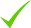IdentityEducation

Know how UrbanPro verifies Tutor details

Identity is verified based on matching the details uploaded by the Tutor with government databases.

## Overview

Ritam R. describes himself as IIT JEE Coach. He conducts classes in Class 11 Tuition, Class 12 Tuition and Engineering Entrance Coaching. Ritam is located in Sakinaka East, Mumbai. Ritam takes at students Home, Regular Classes- at his Home and Online Classes- via online medium. Ritam has completed Bachelor of Technology (B.Tech.) from Indian Institute of Technology. He is well versed in Hindi, English and Bengali.

Hindi

English

Bengali

## Education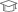Indian Institute of Technology

Bachelor of Technology (B.Tech.)

Sakinaka East, Mumbai, India - 400072

## Verified Info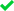Phone VerifiedEmail Verified

Report this Profile

Is this listing inaccurate or duplicate? Any other problem?

Type the letters as shown below *

Please enter the letters as show below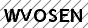Engineering Entrance Coaching classes

Class LocationOnline (video chat via skype, google hangout etc)Student's HomeTutor's Home

## Reviews

this is test message this is test message this is test message this is test message this is test message this is test message this is test message

No Reviews yet! Be the first one to Review

## FAQs

1. Which classes do you teach?

I teach Class 11 Tuition, Class 12 Tuition, Engineering Entrance Coaching and MBA Entrance Coaching Classes.

2. Do you provide a demo class?

Yes, I provide a free demo class.

3. How many years of experience do you have?

I have been teaching for less than a year.

## Answers by Ritam R. (1)

Hello Archit, Please see the solutions below: 2. Let (h,k) be the midpoint of the chord. Then the equation of the chord with midpoint (h,k) is: (hx/3)-(ky/7) = (h^2/3) - (k^2/7) (Equation given by T1 = S1). 7x + y = 20 is the equation of the same line. Comparing the coefficients of the line... ...more
Hello Archit, Please see the solutions below: 2. Let (h,k) be the midpoint of the chord. Then the equation of the chord with midpoint (h,k) is: (hx/3)-(ky/7) = (h^2/3) - (k^2/7) (Equation given by T1 = S1). 7x + y = 20 is the equation of the same line. Comparing the coefficients of the line we get: h/(3*7) = -k/(7) = ((h^2/3) - (k^2/7))/20 So k/h = -1/3 Now the line whose equation we require to find passes through the origin and (h,k) So the equation of the line is y = (k/h).x Since k/h = -1/3, the equation of the line is y = (-1/3)x ---------------------------------------------------------------------------------------------------------------------------------------------- 3. The equation of the hyperbola can be written as (x^2/16) - (y^2/25) = 1 Let the point on the Hyperbola be (4secA, 5 tan A) The equations of the assymptotes are given by (x^2/16) - (y^2/25) = 0 Factorizing out the 2 stratight lines, we get the 2 equations as y = 5x/4 and y = -5x/4 Let Q be the point on y = 5x/4. Since the abscissa remains same, the x coordinate of Q is 4secA. So the y coordinate of Q is y = 5.4secA/4 = 5secA So Q: (4secA, 5secA). Now R lies on y = -5x/4. Solving similarly like we did for Q, the coordinates of R would be (4secA, -5secA). We also have P: (4secA,5tanA) So, PQ = 5(secA-tanA) and PR = 5(secA+tanA) Therefore, PQ.PR = 25.((secA)^2-(tanA)^2) For any angle A, ((secA)^2-(tanA)^2) = 1 Therefore, PQ.PR = 25. Let me know if you have any questions. Thanks
Dislike Bookmark
Engineering Entrance Coaching classes 2.5

Class LocationOnline (video chat via skype, google hangout etc)Student's HomeTutor's Home

MBA Entrance Coaching classes 2.5

Class Location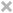Online (video chat via skype, google hangout etc)Student's HomeTutor's Home

Class 11 Tuition 3.2

Class LocationOnline (video chat via skype, google hangout etc)Student's HomeTutor's Home

Board

CBSE

CBSE Subjects taught

Mathematics

Taught in School or College

No

Class 12 Tuition 3.2

Class LocationOnline (video chat via skype, google hangout etc)Student's HomeTutor's Home

Board

CBSE

CBSE Subjects taught

Mathematics

Taught in School or College

No

this is test message this is test message this is test message this is test message this is test message this is test message this is test message

No Reviews yet! Be the first one to Review

## Answers by Ritam R. (1)

Hello Archit, Please see the solutions below: 2. Let (h,k) be the midpoint of the chord. Then the equation of the chord with midpoint (h,k) is: (hx/3)-(ky/7) = (h^2/3) - (k^2/7) (Equation given by T1 = S1). 7x + y = 20 is the equation of the same line. Comparing the coefficients of the line... ...more
Hello Archit, Please see the solutions below: 2. Let (h,k) be the midpoint of the chord. Then the equation of the chord with midpoint (h,k) is: (hx/3)-(ky/7) = (h^2/3) - (k^2/7) (Equation given by T1 = S1). 7x + y = 20 is the equation of the same line. Comparing the coefficients of the line we get: h/(3*7) = -k/(7) = ((h^2/3) - (k^2/7))/20 So k/h = -1/3 Now the line whose equation we require to find passes through the origin and (h,k) So the equation of the line is y = (k/h).x Since k/h = -1/3, the equation of the line is y = (-1/3)x ---------------------------------------------------------------------------------------------------------------------------------------------- 3. The equation of the hyperbola can be written as (x^2/16) - (y^2/25) = 1 Let the point on the Hyperbola be (4secA, 5 tan A) The equations of the assymptotes are given by (x^2/16) - (y^2/25) = 0 Factorizing out the 2 stratight lines, we get the 2 equations as y = 5x/4 and y = -5x/4 Let Q be the point on y = 5x/4. Since the abscissa remains same, the x coordinate of Q is 4secA. So the y coordinate of Q is y = 5.4secA/4 = 5secA So Q: (4secA, 5secA). Now R lies on y = -5x/4. Solving similarly like we did for Q, the coordinates of R would be (4secA, -5secA). We also have P: (4secA,5tanA) So, PQ = 5(secA-tanA) and PR = 5(secA+tanA) Therefore, PQ.PR = 25.((secA)^2-(tanA)^2) For any angle A, ((secA)^2-(tanA)^2) = 1 Therefore, PQ.PR = 25. Let me know if you have any questions. Thanks
Dislike Bookmark

Ritam R. describes himself as IIT JEE Coach. He conducts classes in Class 11 Tuition, Class 12 Tuition and Engineering Entrance Coaching. Ritam is located in Sakinaka East, Mumbai. Ritam takes at students Home, Regular Classes- at his Home and Online Classes- via online medium. Ritam has completed Bachelor of Technology (B.Tech.) from Indian Institute of Technology. He is well versed in Hindi, English and Bengali.

•• Want to learn from Ritam R.?

• Contact Now
X

Recommended Profiles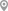Antop Hill, MumbaiGoregaon East, MumbaiUttam Nagar, DelhiMira-Bhayandar, MumbaiArjun Nagar, GurgaonC V Raman Nagar, Bangalore

X

Let us shortlist and give the best tutors and institutes.

or

Send Enquiry to Ritam R.

Let Ritam R. know you are interested in their class